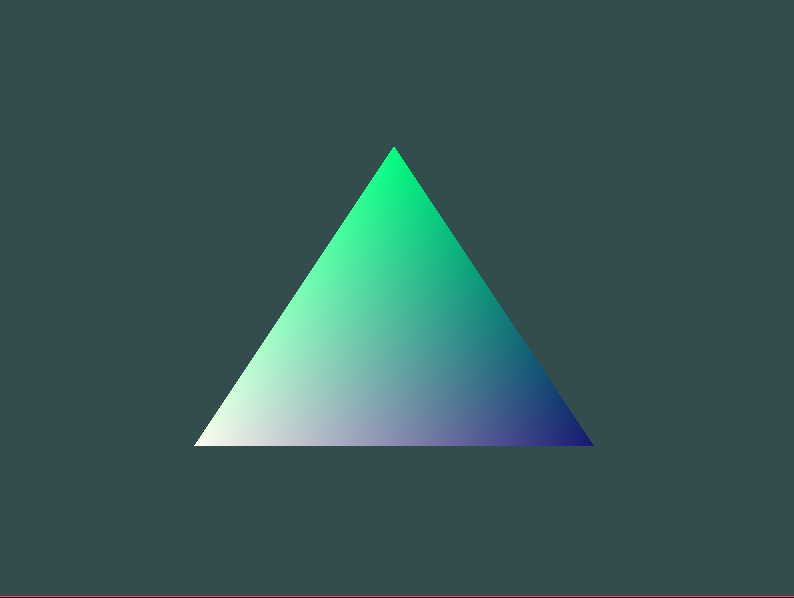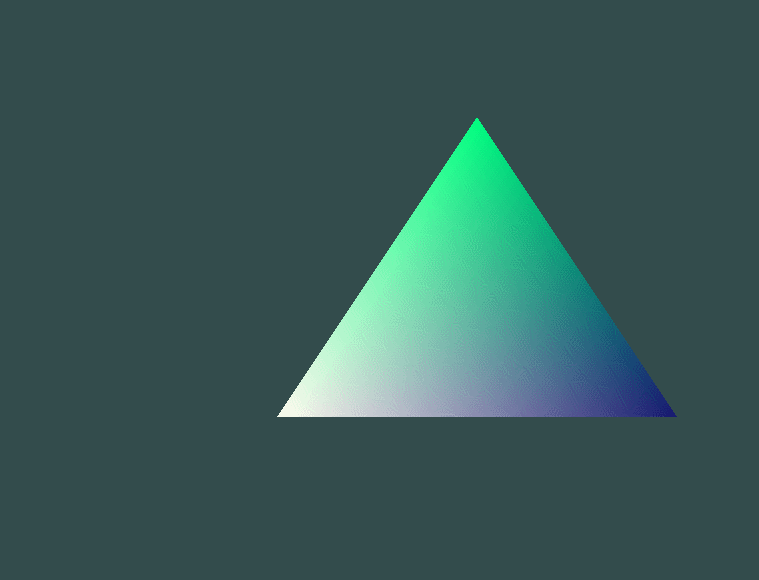We last left our game project with a console app that takes input. However, a console app isn’t going to cut it for the game we want to make. It doesn’t give us enough control of the either the display or access to the mouse. So we need graphics.

OpenTk seems like a pretty good choice. It has a maintained nuget package for C#, which will work in our F# project with minimal fuss. Also, the fact that it is basically a wrapper around OpenGL means that we can reference all the OpenGL resources, of which Learn OpenGL seems really good.

So let’s get something on the screen!

So there are three things we need to do to accomplish this:

1) Add shaders to the project, which OpenGL uses to transform data. 2) Add the OpenTk/OpenGL code to the project that will load the shaders and handle the graphics 3) Integrate our “game” logic with the OpenTk/OpenGL code

There are two shaders that we need a vertex shader and a fragment shader. The vertex shader creates gl_Positions from its input and the fragment shaders deals with colors.

The contents of the vertexShader.vert file are as follows:

#version 440 core

layout (location = 0) in vec3 aPos;
layout (location = 1) in vec3 aColor;

out vec3 ourColor;

void main()
{
gl_Position = vec4(aPos, 1.0);
ourColor = aColor;
}

#version 440 core

in vec3 ourColor;
out vec4 FragColor;

void main()
{
FragColor = vec4(ourColor, 1.0);
}

For more information on what is going on here, take a look at the shader post on Learn OpenGL. Basically, shaders are little programs that run on the GPU written in a C-like language called GLSL.

One last task before we are done with the shaders, we need to add them to the build.

Open build.fsx file. Than add two new directory variables.

let componentsDirSource = "./components/"
let componentsDirDest = buildDir + "components/"

Next modify the build command to copy the components to the output directly.

Target "Build" (fun _ ->
// compile all projects below src/app/
MSBuildDebug buildDir "Build" appReferences
|> Log "AppBuild-Output: "

CopyDir (directory componentsDirDest) componentsDirSource allFiles
)

Now when we run FAKE:Build the shader files are copied to the output folder as expected, where the resulting exe can load them.

OpenGL object

Now the shader files exist, but we still aren’t doing anything with them. Let’s fix that.

OpenTk is a C# library so it isn’t really setup for a functional program. For now, I am not going to worry about that to much. But later (another blog post later), I want to figure out a good way to encapsulate the non-function stuff and add a functional/non-mutable api on top of it. For now, we need to create a class that inherits from GameWindow.

type MyGameWindow() as this =
inherit GameWindow(800, 600, GraphicsMode.Default, "Look! I am an application title!")

do this.Resize.Add(fun e -> GL.Viewport(0, 0, this.Width, this.Height))

this.VSync <- VSyncMode.On
)

do this.UpdateFrame.Add(fun e -> //game code goes here
)

member this._RenderFrameEvent e =

GL.ClearColor(0.2f, 0.3f, 0.3f, 1.0f)

this.SwapBuffers()

So what is going on here? We are creating a GameWindow object with a hard coded width of 800 and height of 600. It has a couple of event handlers defined, for Resize, Load, UpdateFrame, and RenderFrame. Right now nothing much happens in those event handlers, but we will need to add the game update code in UpdateFrame (it basically replaces the game loop we made last time) and the drawing code in RenderFrame. If we put to much stuff in the UpdateFrame handler we should copy what was done for the RenderFrame and add a real method that the handler calls, but that is more of a style choice.

Anyway, let’s replace the game loop we had with the OpenTk game loop.

/// <summary>
/// The main entry point for the application.
/// </summary>
[<EntryPoint>]
let main argv =
//    let initialState =
//            {
//                shouldContinue = true;
//                position = 0.0f, 0.0f;
//            }
let gw = new MyGameWindow()
do gw.Run(60.0)
0

I will come back to the currently unused initialState, but all this does is create an instance of the MyGameWindow class and call its inherited Run method with an update rate limit specified.

Creating the Program

Now that we have the framework in place, let’s load the shaders and use them. To do this we need to read the shaders from the files, compile them, and put them in a program that we can use when drawing things. For now, we can create a method on the MyGameWindow class called CompileProgram that will do all these things for us, and return the id for the program so we can use it later.

member this.CompileProgram () =
let currentDirectory = Environment.CurrentDirectory

//print shader compilation errors to the console

//print shader compilation errors to the console

let program = GL.CreateProgram()

//print program errors to the console
let programResults = GL.GetProgramInfoLog(program)
printf "%s" programResults

{
ProgramId = program;
}

Now we can call this method in the Load event handler.

this.VSync <- VSyncMode.On
this.Ids <- this.CompileProgram()
)

CompileProgram doesn’t just return the ProgramId it also returns the two shader Ids. This is two facilitate clean up in the Exit event handler. We will need to define a type to hold all three ids as well as add the Exit event handler.

type OpenGlIds = {
ProgramId: int;
VertexId: int;
FragmentId: int;
}
override this.Exit () =
GL.DeleteProgram(this.Ids.ProgramId)
base.Exit()

member val Ids: OpenGlIds = { ProgramId = 0; VertexId = 0; FragmentId = 0; } with get,set

Next, we need data to pass OpenGL, in a form that it understands. To do this we need a Buffer (VBO) that will hold the data and a VertexArray (VAO) that describes the data format to OpenGL. Similar to the shader setup we will create a method to create the objects that returns the ids for later cleanup. However, there is also an additional method to load data into the buffer. This additional method, LoadData is what will get called in the UpdateFrame handler.

type BufferData = {
Vbo: uint32;
Vao: uint32;
}
member val Buffers: BufferData = { Vbo = 0u; Vao = 0u; } with get,set
member this.CreateBuffers() =
let mutable vbo = 0u
let mutable vao = 0u

GL.GenVertexArrays(1, &vao)
GL.GenBuffers(1, &vbo)

{
Vbo = vbo;
Vao = vao;
}

let vao = this.Buffers.Vao
let vbo = this.Buffers.Vbo

GL.BindVertexArray(vao)
GL.BindBuffer(BufferTarget.ArrayBuffer, vbo)

GL.BufferData(BufferTarget.ArrayBuffer, IntPtr(vertices.Length * Vector3.SizeInBytes), vertices, BufferUsageHint.StaticDraw)

GL.VertexAttribPointer(0, 3, VertexAttribPointerType.Float, false, 2 * Vector3.SizeInBytes, 0)
GL.EnableVertexAttribArray(0)

GL.VertexAttribPointer(1, 3, VertexAttribPointerType.Float, false, 2 * Vector3.SizeInBytes, Vector3.SizeInBytes)
GL.EnableVertexAttribArray(1)

//unbind
GL.BindBuffer(BufferTarget.ArrayBuffer, 0)
GL.BindVertexArray(0)

vao
this.VSync <- VSyncMode.On
this.Ids <- this.CompileProgram()
this.Buffers <- this.CreateBuffers()
)

Now let’s update the UpdateFrame method to load data into the buffer by calling LoadData.

First we need to add a property to hold the id of the VAO to use when drawing.

member val DrawingData: uint32 = 0u with get,set

Then, define the vertices and put the resulting VAO into that property.

let vertices =
[| //Postion                            | Colors
Vector3(-0.5f, -0.5f, 0.0f);  Vector3(1.00f, 1.00f, 0.94f);
Vector3( 0.5f, -0.5f, 0.0f);  Vector3(0.09f, 0.09f, 0.44f);
Vector3( 0.0f,  0.5f, 0.0f);  Vector3(0.00f, 1.00f, 0.50f);
|]

Drawing The Triangle

One last step, actually drawing the triangle. Add the following three lines to the RenderFrameEvent method.

GL.UseProgram(this.Ids.ProgramId)
GL.BindVertexArray(this.DrawingData)
GL.DrawArrays(PrimitiveType.Triangles, 0, 3)

This tells OpenGL to use the program with the attached shaders, binds the VertexArray with the data, and than tells it to use the first three of the data points to draw triangles.

Run It!

Run FAKE: Build to build the project and than run the exe in the build folder of the project. The result should look like this:User Input

So that was a bit of a trek, but now we have something on the screen again. Still, we lost some functionality, specific user input. We can do that pretty easier at this point.

We can start by defining a game state type.

type GameState =
{
shouldContinue: bool;
position: float32*float32;
}

We are going to use the position parameter, which holds an (x,y) coordinate tuple, to move the triangle around. The shouldContinue bool behaves like it did in part one. We can define a couple methods to accomplish this.

let updatePosition (dx,dy) (x,y) =
x+dx, y+dy

let move delta state =
{ state with position = updatePosition delta state.position }

let deltaX = 0.1f
let deltaY = 0.1f

let moveRight state =
move (deltaX, 0.0f) state

let moveLeft state =
move (-1.0f * deltaX, 0.0f) state

let moveUp state =
move (0.0f, deltaY) state

let moveDown state =
move (0.0f, -1.0f * deltaY) state

let exitGame gameState =
{gameState with shouldContinue = false}

Now we need to call these based on user input. A function that takes the keyboard state and the current game state and returns the new game state seems like a good starting point.

let updateState (keyState: KeyboardState) gameState =
match keyState with
| ks when ks.IsKeyDown(Key.Escape) -> exitGame gameState
| ks when ks.IsKeyDown(Key.Right) -> moveRight gameState
| ks when ks.IsKeyDown(Key.Left) -> moveLeft gameState
| ks when ks.IsKeyDown(Key.Up) -> moveUp gameState
| ks when ks.IsKeyDown(Key.Down) -> moveDown gameState
| _ -> gameState

Now the MyGameWindow object will need to be updated to have a game state and call updateState in updateFrame. Instead of having it call it directly, we can inject the method in the constructor (along with the initial game state, I told you we would get back to that!).

type MyGameWindow(initialState, onUpdateFrame) as this =
member val GameObject: GameState = initialState with get,set
/// <summary>
/// The main entry point for the application.
/// </summary>
[<EntryPoint>]
let main argv =
let initialState =
{
shouldContinue = true;
position = 0.0f, 0.0f;
}
let gw = new MyGameWindow(initialState, updateState)
do gw.Run(60.0)
0

The final step is to update the game state on UpdateFrame.

let keyState = this.Keyboard.GetState()
this.GameObject <- onUpdateFrame keyState this.GameObject

let x, y = this.GameObject.position

let vertices =
[| //Postion                            | Colors
Vector3(-0.5f + x, -0.5f + y, 0.0f);  Vector3(1.00f, 1.00f, 0.94f);
Vector3( 0.5f + x, -0.5f + y, 0.0f);  Vector3(0.09f, 0.09f, 0.44f);
Vector3( 0.0f + x,  0.5f + y, 0.0f);  Vector3(0.00f, 1.00f, 0.50f);
|]